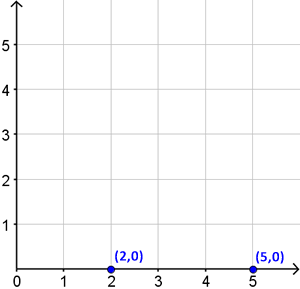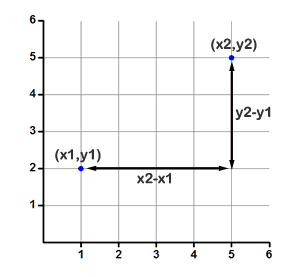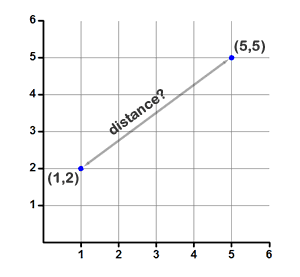# Calculate the Distance Between Two Points

### Introduction

We use what's known as the distance formula to obtain the distance between any two points in space. Suppose you need to determine the distance between the points (2,0) and (5,0). Here's how they look on a graph:With just a quick glance, you can tell they are 3 units apart. They're both on the x axis, so it's just a straight line measurement. In fact, you may not have even needed the graph at all to figure that one out. But, how can you measure the distance between two random points on a graph? Why, use the distance formula of course!

$$\text{distance}= \sqrt{\big(x_{2}-x_{1}\big)^2+\big(y_{2}-y_{1}\big)^2}$$

### Breaking it down

At first glance, that formula looks like a real mess! But just think about the $$x_{2}-x_{1}$$ and $$y_{2}-y_{1}$$ components as the length in each direction. Using those two values we mathematically construct an imaginary triangle with two legs whose length we can calculate. Let me show you what I mean visually:We're just measuring the distance along each axis, and then using the pythagorean theorem to compute the length of the hypotenuse, which is the imaginary line directly between our two points. It doesn't matter which point is $$(x_{1},y_{1})$$ and which is $$(x_{2},y_{2})$$. The key idea to take away from this plot is that you only care about the change in x and the change in y. You'll use each of those measurements as the side of a triangle, where the hypotenuse is the distance between the two points. You could express the distance formula like this:

$$\text{distance}= \sqrt{\big(\text{change in x}\big)^2+\big(\text{change in y}\big)^2}$$

How did we come up with that formula? It's simply the pythagorean theorem, which allows us to find the hypotenuse of a right triangle. In our case, the hypotenuse is the distance between the two points!

Let's look at a simple example now:

### Example:

Find the distance between the two points (5,5) and (1,2) by using the distance formula.

Solution:

Rather than blindly plugging numbers into a formula, draw a graph so you know what's going on.Those are your two points. Remember that the important values are the change in x and the change in y. That's equivalent to saying, what is $$x_2-x_1$$ and what is $$y_2-y_1$$.

Here, the change in x is 4 units, and the change in y is 3 units. You can even count the lines on the graph to make sure that's correct.

$$\text{distance}= \sqrt{\big(4\big)^2+\big(3\big)^2}$$ $$\text{distance}= \sqrt{16+9}$$ $$\text{distance}= \sqrt{25}$$ $$\text{distance}= 5$$

Once we knew that $$x_{2}-x_{1}$$ was 4 and $$y_{2}-y_{1}$$ was 3, we just inserted those numbers into the distance formula to solve.

You might be wondering what happens if you reverse the points? Remember that we are dealing with distances, which are inherently positive. The distance is the same in either direction, going from point 1 to point 2 or vice versa. So, just use the positive distance between two points. If you look at the formula, you'll notice that $$x_{2}-x_{1}$$ and $$y_{2}-y_{1}$$ are squared, which automatically makes them positive anyway. So it doesn't matter which point is which -- you'll still get a positive distance!

Let's look at another example and solve it without using a graph:

### Example:

Find the distance between the two points (8,-2) and (3, 9).

Solution:

Now that you understand how the distance formula works, you can plug the numbers straight into the formula:

$$\text{distance}= \sqrt{\big(8-3\big)^2+\big(-2-9\big)^2}$$ $$\text{distance}= \sqrt{25+121}$$ $$\text{distance}= \sqrt{146}$$ $$\text{distance}= 12.08$$

The distance formula is not complicated -- you just need to practice with a graph so you understand what's going on. You're simply making a triangle and finding the length of the hypotenuse. The distance formula is just the pythagorean theorem!

### Extending to three-dimensions

What about a three-dimensional space? How would we find the distance between the points (1,5,0) and (2,0, 8)? It's certainly a lot harder to draw a graph and measure the distance! The three-dimensional distance formula is actually super simple. Just add another term into the formula because we have to account for the z-axis now.

$$\text{distance}= \sqrt{\big(x_{1}-x_{2}\big)^2+\big(y_{2}-y_{1}\big)^2+\big(z_{2}-z_{1}\big)^2}$$

If you've made it this far, hopefully you have a better understanding of the distance formula. If that's not the case, here's another distance formula lesson available online.